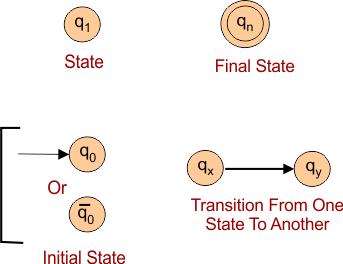Select Page

# Theory of Automata

Theory of automata is the study of abstract machines and the computation problems. The computation problems can be solved using these abstract machines. The abstract machine is called the automata or automaton.

Automata consist of states and transitions. The State is represented by circles, and the Transitions are represented by arrows.

Automata takes some input strings as input and this input goes through some states and may enter in the final state.

## Basic Elements of Automata

There are some basic elements of Automata are explained below

### 1. Symbols

Symbols involve letter, alphabet or any picture.

Example: 1, a, b, #

### 2. Sigma (∑)

Sigma contains a finite set of symbols. It is also known as Alphabets and denoted by ∑.

Examples: ∑ = {a, b} , ∑ = {A, B, C, D} , ∑ = {0, 1, ….., 5]

### 3.Grammar

It consist of Set of production Rules. it is mostly denoted by P.

Example: Production P : S → aAb, aA → aaAb, A → ε. Where S → aAb is one the production rule.

### 4. Language

Production rules of grammar are used to create the set of Strings. Collection of these strings is called Language. A language which is formed over Sigma (Σ) can be Finite or Infinite.

Example: 01

Language (L) = {Set of string of length 2} = {xx, yy, xy, yx}        //  Finite Language

Example: 02

L2 = {Set of all strings starts with ‘x’} = {x, xx, x, xyy, xyyyy, xyxyxyyyx………}      //  Infinite Language

### 5. String

It is collection of some symbols from the Sigma. The string is denoted by w.

Example: If ∑ = {x, y}, then various string that can be generated from ∑ are {x, y, xxx, xyxy, yy, xyy, …..}.

• A string with ‘0’ occurrences of symbols is called empty or epsilon string. It is denoted by ε.
• The total number of symbols in a string (w) is called the length of that string. It is denoted by |w|. if the string is w= 010 then it means its length is 3.

## Notations in AutomataImportant: Languages are generated according to its grammar and accepted or rejected by automat. Automata are abstract machine which accept or reject the regular languages.

Note: All regular languages are represented with Regular Expressions

Help Other’s By Sharing…